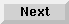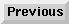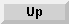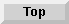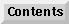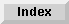5 Operators and Functions

## Built-in Numeric Functions

A function is a routine that manipulates numeric or string data and produces a numeric or string value as a result. A set of commonly used functions, such as one to compute the square root of a number, is supplied as part of the Eloquence language. These functions are known as built-in functions. The built-in functions that perform standard numeric routines are:

ABS (X)
Absolute value of X.
BACKGROUND
Returns 1 if executing in background (stdout redirected or -b switch on commandline). Implies NO OPERATOR. Otherwise returns 0.
DROUND (X,Y)
Returns the value of X rounded to Y number of digits.
EXP (X)
Naperian e raised to the power X.
FRACT (X)
Returns the fractional part of X.
INT (X)
Largest integer %<= X.
LGT (X)
Logarithm to the base 10 of X; X > 0.
LOG (X)
Natural logarithm; X > 0.
NO OPERATOR
Returns 1 if executing in batch mode (stdin redirected); else 0. E.g., eloq PROG %< infile.
NUMREC file number
Returns the highest used record number of the file ASSIGNED to a file number. If the file number is ASSIGNED to an HP-UX sequential file, this will result in error 58. If this file is being used as a workfile, it will return the same as WFLEN.
PI
Returns the value of $\pi$, 3.14159265359.
PID
Returns process/parent process id.
PNTR
Current 'PRINTER IS'. Returns current printer number, or:
-3

if PRINTER IS "SPOOLFILE"

-6

if PRINTER IS "STDOUT"

-7

if PRINTER IS "STDERR"

-8

if PRINTER IS "TTY"

-9

if PRINTER IS "CONSOLE"

PPID
Returns parent process id.
PROUND (X,Y)
Returns the number X rounded to the power of 10 position specified by Y.
RND
Returns a pseudo-random number in a standard sequence of numbers > 0 and < 1.
RANDOMIZE (start value)
This will reset the (pseudo-) random number generator to a new starting value. If the start value is omitted, random number generator starting value is derived from current system time. If the optional start value is given, it will first be converted to an integer value and then the lower 48 bits of it will be used to initialize random number generation.
SGN (X)
Returns -1 if X is negative, 0 if X is 0, and 1 if X is positive.
SQR (X)
Returns the square root of X.
MAX (list)
Returns the highest value in the list of numeric expressions.
MIN (list)
Returns the lowest value in the list of numeric expressions.
USRID
Returns the user id number. This is calculated using the HP-UX function tty slot(). The following program in C displays this slot # for the terminal where you execute the program:
                main() {
int ttyslot () ;
printf ("ttyslot: %d\\n",ttyslot());
}

CURKEY
Returns the number of the most recently pressed softkey or the value of an ONCONDITION interrupt if TIO is used.
Some functions are available for compatibility reasons, only. To get the syntax of this functions,see section , Built-in Numeric Function, on page 3

NOTE: There is a built-in string function called REVISION\$ (not to be confused with REVISION) that returns the version of Eloquence currently running, as a seven-character string. For example, A.06.00.

Built-in numeric functions usually consist of a function name followed by one parameter. The parameter may be a number (as in line 10 below), a numeric variable (line 20), or a numeric expression (line 30). Since the result of a numeric function is always a single value, a numeric function can be used as an operand in an expression (line 40) or as a parameter of a numeric function (line 50). Here are some examples:

10   A=INT(1.6)     A equals the integer value of 1.6 = 1.
20   B=ABS(A)       B equals the absolute value of 1 = 1.
30   C=SQR (A+B)    C equals the square root of (1 + 1) = 1.41421356237.
40   D=LGT(A)^2+LOG(A)^2

D equals the sum of LGT(A) squared plus LOG(A) squared (0).


50   E=INT(SQR(10))      SQR(10) = 3.14; the integer value of 3.14 = 3.

60   Max=MAX(1,35,7)     Max = the largest value in the list = 35.
70   R=PROUND(125.2,2)   125.2 rounded to the second power of ten = 100.
80   PI=DROUND(PI,4)     PI rounded to four digits = 3.142.


The last three lines show built-in functions which allow more than one parameter.

Other built-in functions are available to manipulate strings, to return information on file storage operations, and to control the format of program output. In addition, you may define your own functions as explained later in this chapter.

### Trigonometric Statements and Functions

The trigonometric functions use one of three angular units: radians, degrees, or gradients (grads). Radians are the default units (set at power-up or after the computer is reset). To change the angular units, execute one of these statements:

DEG
sets degees units. A degree is 1/360th of a circle.
The trigonometric functions available are:

ACS (X)
returns the principal value of the arccosine of the numeric expression X, which can range from -1 to +1.
ASN (X)
returns the principal value of the arcsine o the numeric expression X, which can range from -1 to +1.
ATN (X)
returns the principal value of the arctangent of the numeric expression X.
COS (X)
returns the cosine of the angle represented by the numeric expression X.
SIN (X)
returns the sine of the angle represented by the numeric expression X.
TAN (X)
returns the tangent of the angle represented by the numeric expression X.
Here are some examples of trigonometric operations:

       DEG
FIXED5
DISP SIN(60)
.86603
COS(45)
.70711
ASN(.5)
30.00000
ACS(.5)
60.00000
ATN(.5)
26.56505

FIXED5
DISP SIN(PI/6)
.50000
COS(PI/6)
.86603
TAN(PI/4)
1.00000
ASN(.5)
.52360
ACS(.5)
1.04720
ATN(.5)
.46365

FIXED5
DISP SIN(-70)
-.89101
COS(-70)
.45399
TAN(50)
1.00000
ASN(.5)
33.33333
ACS(.5)
66.66667
ATN(.5)
29.51672

NOTE: To use the SIN function you need to precede it with DISP otherwise it conflicts with space independent (SI) and causes an error message.

### Random Numbers

A random number generator, the RND function, is provided for programs that perform simulations. Each time the RND function is called, a random number between 0.0 and 1.0 is returned. The following program demonstrates the RND function:


10   FIXED 12
20   FOR Line = 1 TO 10
30     PRINT RND,RND,RND,RND
40   NEXT Line
50   END


Eloquence Language Manual - 19 DEC 2002